# PSEB 5th Class Maths Solutions Chapter 1 Numbers Ex 1.2

Punjab State Board PSEB 5th Class Maths Book Solutions Chapter 1 Numbers Ex 1.2 Textbook Exercise Questions and Answers.

## PSEB Solutions for Class 5 Maths Chapter 1 Numbers Ex 1.2

Question 1.
Write the place value of underlined digits in the following numbers :
(a) 8326
(b) 54588
(c) 90008
(d) 99234
(e) 49716
(f) 47168
(g) 63184
(i) 29999.
Solution:
(a) 8326 The place value of underlined digit i.e. 2 = 2 × 10 = 20
(b) 54588 The place value of underlined digit i.e. 4 = 4 × 1000 = 4000
(c) 90008 The place value of underlined digit i.e. 8 = 8 × 1 = 8
(d) 99234 The place value of underlined digit i.e. 9 = 9 × 10000 = 90000
(e) 49716 The place value of underlined digit i.e. 7 = 7 × 100 = 700
(f) 47168 The place value of underlined digit i.e. 7 = 7 × 1000 = 7000
(g) 63184 The place value of underlined digit i.e. 6 = 6 × 10000 = 60000
(h) 29999 The place value of underlined digit i.e. 2 = 2 × 10000 = 20000

Question 2.
Write the face value of underlined digits in the following numbers :
(a) 82232
(b) 54180
(c) 90811
(d) 12994
(e) 11973
(f) 24716
(g) 11631
(h) 59999.
Solution:
(a) 82232 The face value of underlined digit i.e. 2 = 2
(b) 54180 The face value of underlined digit i.e. 4 = 4
(c) 90811 The face value of underlined digit i.e. 8=8
(d) 12994 The face value of underlined digit i.e. 9=9
(e) 11973 The face value of underlined digit i.e. 7 = 7
(f) 24716 The face value of underlined digit i.e. 7=7
(g) 11631 The face value of underlined digit i.e. 6 = 6
(h) 59999 The face value of underlined , digit i.e. 5 = 5Question 3.
Write the following numbers in the expanded form :
(a) 232
(b) 4180
(c) 27811
(d) 82994
(e) 10073
(f) 43710
(g) 61639
(h) 26999.
Solution:
(a)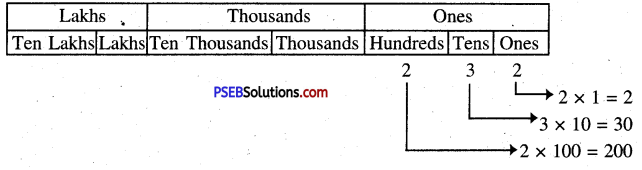The expanded form of 232 = 200 + 30 + 2

(b)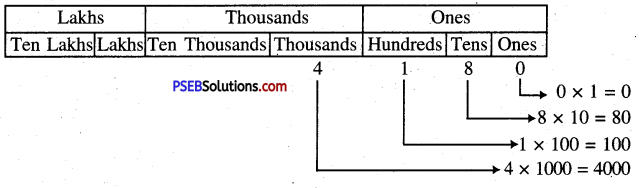The expanded form of 4180 = 4000 + 100 + 80 + 0

(c)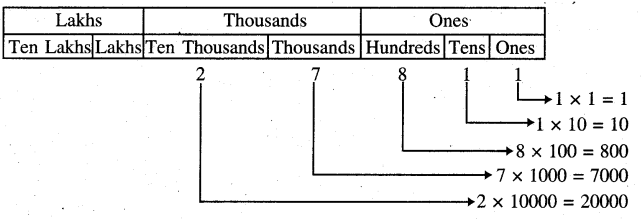The expanded form of 27811 = 20000 + 7000 + 800 + 10 + 1

(d)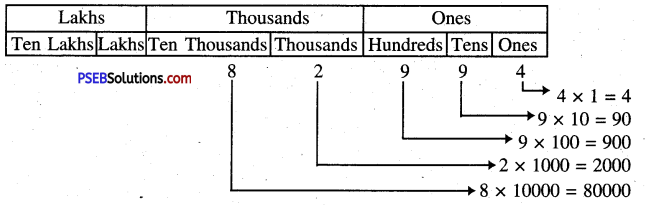The expanded form of 82994 = 80000 + 2000 + 900 + 90 + 4(e)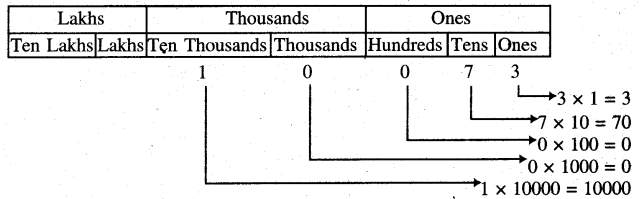The expanded form of 10073 = 10000 + 70 + 3

(f)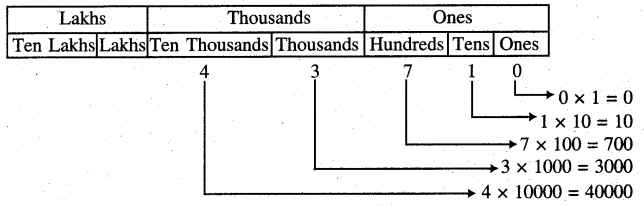The expanded form of 43710 = 40000 + 3000 + 10

(g)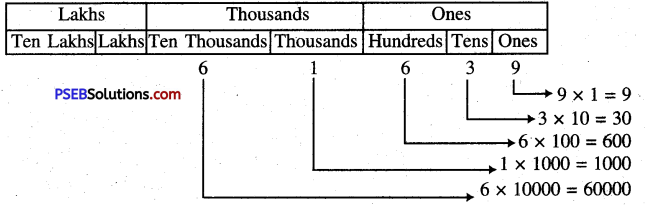The expanded form of 61639 = 60000 + 1000 + 600 + 30 + 9

(h)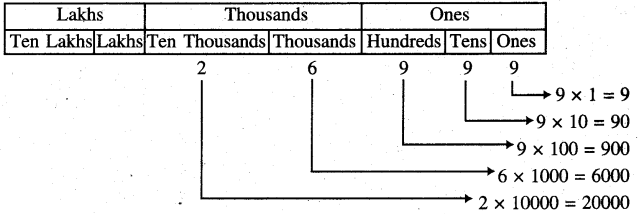The expanded form of 26999 = 20000 + 6000 + 900 + 90 + 9